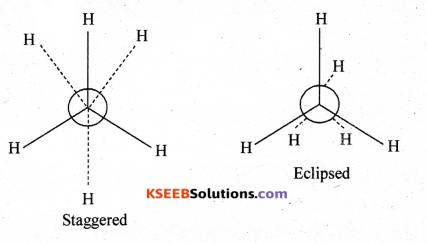# 1st PUC Chemistry Model Question Paper 3 with Answers

Students can Download 1st PUC Chemistry Model Question Paper 3 with Answers, Karnataka 1st PUC Chemistry Model Question Papers with Answers helps you to revise the complete Karnataka State Board Syllabus and score more marks in your examinations.

## Karnataka 1st PUC Chemistry Model Question Paper 3 with Answers

Time: 3.15 Hours
Max Marks: 70

Instruction:

1. The questions paper has five parts A, B, C, D and E. All parts are compulsory.
2. Write balanced chemical equations and draw labeled diagram wherever allowed.
3. Use log tables and simple calculations f necessary (use of scientific calculations is not allowed).

Part – A

I. Answer ALL of the following (each question carries one mark): ( 10 × 1 = 10 )

Question 1.
‘Cis platin’ a medicine used in the treatment of which disease?
Cancer/Cancer Tumour.

Question 2.
Write the mathematical expression for Boyle’s law.
V ∝ $$\frac{1}{P}$$ at constant temperature.

Question 3.
Give the example for gaseous reversible reaction for which Kp = Kc
H2(g) + I2(g) ⇌ 2HI(g)

Question 4.
Which group elements in the periodic table are called Noble gases?
18th group.Question 5.
What is the oxidation number of the element in its free state?
0 or Zero.

Question 6.
Write the general electronic configuration of First group elements.
ns1

Question 7.
Why is boric acid considered as weak acid?
Due to small size of B and presence of only six electrons in the valence shell i.e. protonic acid.

Question 8.
Mention the structure of SiO44-.
Ans.
Tetrahedral structure.Question 9.
Give an example for non-benzenoid compound.
Pyridine

Question 10.
Write the expanded form of ‘CNG

Part – B

II. Answer any FIVE of the following questions carrying TWO marks ( 5 X 2 = 10 )

Question 11.
Convert 37°C to °F.
°F = $$\frac{9}{5}$$ + °C +32 = $$\frac{9}{5}$$ X 37 + 32 = 98.6°

Question 12.
Under what conditions of temperature and pressure real gases tend to behave ideally?
Low pressure and high temperature.Question 13.
What is dipole moment? What is its SI unit.
It is the product of +ve or negative charge and the distance between the centre of them, μ = e x d
SI unit coulomb meter or cm.

Question 14.
Write any two diagonal relationship between Beryllium and Aluminium.
(a) Both forms covalent compounds and soluble in organic compounds.
(b) Both BeCl2 and AlCl3 act as Lewis acids.

Question 15.
Give the reaction for the synthesis of water gas and producer gas.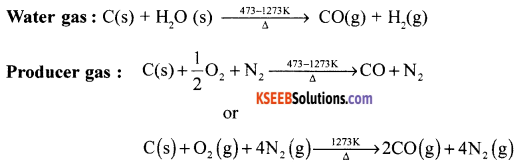Question 16.
How is chloromethane converted to methane?
Chloromethane heated with zinc and NaOH to methane.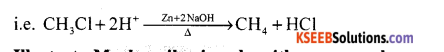Question 17.
Illustrate Markovnikov’s rule with an example.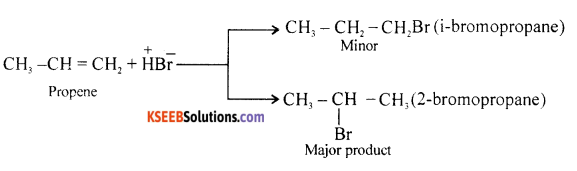The Br of HBr is added to double bonded carbon as containing less of OH atoms to give 2–bromopropanol.

Question 18.
What is BOD? What is its significance?
The amount of oxygen consumed by the microorganisms in decomposing the organic matter present in water is called BOD. It is a water quality parameter to know the amount of organic matter available for bacteria.Part – C

III. Answer any FIVE of the following questions; carrying THREE marks: ( 5 x 3 = 15 )

Question 19.
(a) Give reason : Ionic radius of Fis more than atomic radius of F.
Due to more number of electrons in F than F.

(b) How does ionization enthalpy varies down the group?
Ionisation enthalpy decreases down the group due to increase in atomic size of atoms.

(c) Ionization enthalpy of nitrogen is more than that of oxygen. Give reason.
Due to completely half filled p-orbitals (1s2 2s2 2p3) in nitrogen but in oxygen atom p-orbital is more than half-filled.

Question 20.
With the help of MOT write the energy level diagram of hydrogen molecule. What is its bond order and predict magnetic property.
BO = $$\frac{1}{2}$$ (NBMO NABMO)
= $$\frac{1}{2}$$(2 – 0 ) = 1
It is diamagnetic due to absence of unpaired electrons in the molecule.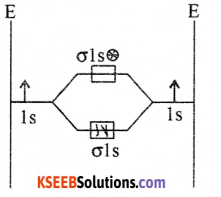Question 21.
Calculate the formal charge of each oxygen atom of ozone molecule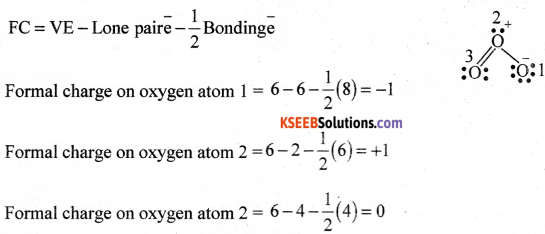Question 22.
(a) Give any two differences between sigma and pi bonds.
Sigma Bond

2. It is strong bond due to large overlapping.
3. Free rotation is possible.

Pi Bond

1. Lateral (sideways) overlapping of AO.
2. It is weak bond due to small overlapping.
3. Free rotation about n bond is not possible.

(b) What is the magnetic nature of oxygen molecule?
It is paramagnetic due to presence of unpaired electrons in πpx πpy antibonding molecular orbitals.

Question 23.
Balance the following redox reaction using oxidation number method.
MnO2 + Br→ Mn2+ +Br2 + H2O (In Acidic medium)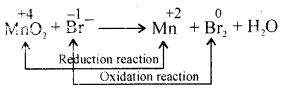Step II – Oxidation Reaction x 2
Reduction Reaction x 1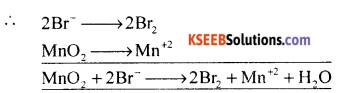Balance the O atoms (add to RHS) and balance the H+ ions (To LHS)
MnO2 + 2B + 4H+ → Mn+2 +2Br2 +2H2O

Question 24.
(i) Explain the laboratory preparation of dihydrogen.
In the laboratory, dihydrogen is prepared by adding dil. H2S04 to granulated zinc.
i.e. Zn + H2SO4 (dil) → ZnSO4 + H2
The H2 gas liberated is collected by downward displacement of water.

(ii) Give an example of ionic hydride.
LiH/NaH/CaH2/BaH2 etc.Question 25.
Give any three points of differences between lithium and other alkali metals.

1. Small size of lithium and its ion.
2. High charge to size ratio (polarising power).
3. High ionization enthalpy and low electropositive character of lithium.
4. Absence of d-orbitals.

Question 26.
(i) Write the molecular formula of silica.
SiO2

(ii) Write the partial structure of silicone.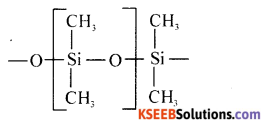(iii) Name the catalyst used in gasoline production.
ZSM-5.

Part – D

IV. Answer any FIV E of the following questions’carrying FIVE marks: ( 5 x 5 = 25 )

Question 27.
(a) An organic compound contains 69% carbon and 4.8% hydrogen, the remainder being oxygen. Calculate the masses of carbon dioxide and water produced when 0.20 gram of this substance is subjected to complete combustion.
Given % carbon = 69%, H = 4.8%, w = 0.20g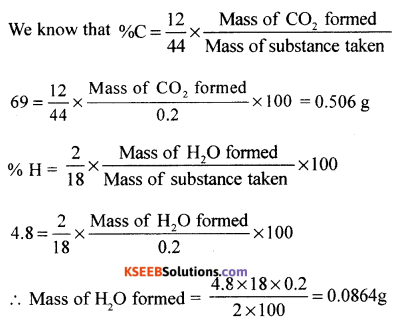(b) Give an example each for element and compound.
Element = sodium/Na.
Compound = NaOH/sodium hydroxide.

Question 28.
(a) Write the significance of quantum numbers n, I and m.
(i) Principal quantum number (n): Determines energy and size of the orbits (electrons).
(ii) Azimuthal Q.N. (1): Determines the shape of the suborbitals (s,p,d,f).
(iii) Magnetic Q.N. (m): Determines the orientation of the orbitals.

(b) State Pauli’s exclusion principle.
For two electrons of an atom the values of n, 1 and m but s is different.
OR
No two electrons of an atom can have the same values of n, 1 and m are same 3 is different.

(c) Write the electronic configuration of copper (Z = 29).
Ans. Cu29= 1s22s22p63s23p63d104s1Question 29.
(a) The FM station of All India Radio, Hassan, broadcast on a frequency of 1020 kilohertz. Calculate the wavelength of the electromagnetic radiation emitted by the transmitter.
Given γ = 1020 kilohertz = 1020 x 1000 hertz
C = 3 x108 ms-11 λ = ?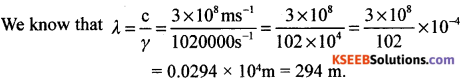(b) What is the maximum number of electrons present in third main energy level?
Third Main energy level = M r /.
∴ No. of electrons in M shell = 18e.

Question 30.
(a) Give any three postulates of kinetic molecular theory of gases.

1. ll gases are made up of with many number of tiny particles called molecules.
2. Gas molecules are separated from each other by large distance. Hence the volume of the gas molecules is negligible compared to total volume of the gas.
3. There is no force of attraction between the gas molecules.
4. The average kinetic energy of gas molecules is directly proportional to Kelvin temperature.

(b) On a ship sailing in Pacific Ocean where temperature is 23.4°C, a balloon is filled with 2L air. What will be the volume of the balloon when the ship reaches 1 the Indian Ocean where temperature is 26.1°C?
V1= 2L V2 = ?
T1= 23.4°C + 273 = 296.4 K
T2 = 26.1°C + 273K = 299.1K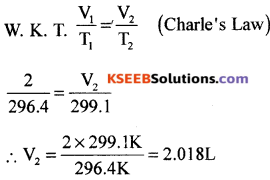Question 31.
(a) The combustion of one mole benzene takes place at 298K and 1 atm. After combustion, CO2(g) and H2O (l) are produced and 3267.0 kJ of beat is liberated. Calculate the standard enthaipy of formation of benzene.
Given : Standard enthalpy of formation of CO2(g) and H2O (1) are – 393.5 kJ mol-1 and -285.0 kJ mol-1 respectively.
Required equation : 6C (s) + 3H2(g) → C6H6 (l); ∆Hf = ?
(i) C6H6(l) + $$\frac{15}{2}$$O2(g) → 6CO2(g) + 3H2O(l); ∆Hc = -3267KJ
(ii) C(s) + O2(g) → CO2 (g); ∆Hf = -393.5 KJ
(iii) H2(s) + $$\frac{1}{2}$$O2(g) → H2O(l); ∆Hf= -285 KJ
reverse the equation (i) + (ii) 6 + (iii) * 3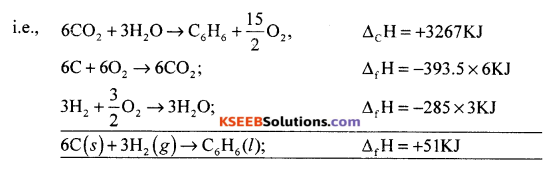(b) Write the mathematical expression for First law of thermodynamics.
∆U = q +w or ∆U = q – P∆V

(c) Give an example of isolated system.
Tea placed in a thermos flask.Question 32.
(a) What are the values for ArH° and ArS° for reaction to be spontaneous at all
temperatures?
When ∆rH° = -ve and ∆rS° = +ve the process is spontaneous at all temperture.

(b) Write the relationship between
(i) Enthalpy (H) and infernal energy (U) (ii) Cp and Cv.
(iii) Free energy (G) Enthalpy (H) and Entropy (S).
(i) ∆H = ∆U + P∆V
(ii) Cp – Cv= R for 1 mole of an ideal gas
Cp – Cv =nR for n moles of an ideal gas
(iii) ∆G = ∆H – T∆S

Question 33.
(a) The following concentrations were obtained for the formation of NH3 from N2 and H2 at equilibrium 500K. [Ni] = 1.5 x 10-2 M, [H2] = 3.0 x 10-2 M and [NHJ = 1.2 x 10-2 m. Calculate the equilibrium constant.
Given [N2] = 1.5 x 10-2 M, [H2] = 3.0 x 10-2 M and [NH3] = 1.2 x 10-2m
For N2(g) + 3H2 (g) → 2NH3 (g)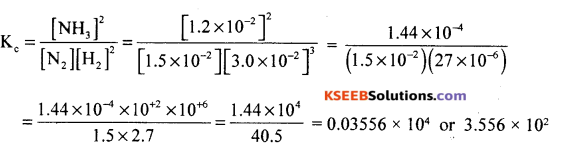b) Define common ion effect
It is the process of decreasing (or suppression) the dissociation of a weak electrolyte by adding a strong electrolyte having a common ion is called common ion effect.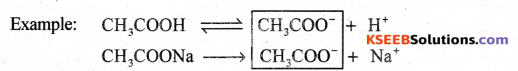(c) What is the relationship between [HsO+] and [OH-] for neutral solution?
[H3O+] = [OH].

Question 34.
(a) What is acid and bases according to Arrhenius concept?
Arrhenius Acid : Substances which give hydrogen ion in water are called Arrhenius Acid. Example: HCl. HNO3
Arrhenius base : Substances which gives hydroxyl ion (OH-) in water are called Arrhenius bases.
Example: NaOH, KQH, NH4OH, etc.

(b) Give an example for (i) so.M-vapoisr equilibrium (ii) liquid-vapour equilibrium,
(i) I2(s) → I2 (vapour) / NH4Cl(s)⇌ NH4Cl(vapour) (ii) H2O(l) → H2O(v).

(c) Write the equilibrium K. expression for H2 + I2
Kc = $$\frac{\left[\mathrm{H}_{2}\right]\left[\mathrm{I}_{2}\right]}{[\mathrm{HI}]^{2}}$$Part – E

V. Answer any TWO of the following questions carrying FIVE marks: ( 2 x 5 = 10 )

Question 35.
(a) For the molecule CH3CH2CH2OH
(i) Identify functional group
Functional group = – OH

(ii) Write the bond line forarulbu
Bond line formula =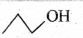(iii) Write the succeeding homologue.
Succeeding homologue = CH3CH2CH2CH2OH

(b) Explain inductive effect with a suitable example.
The permanent displacement of a (sigma) electrons along the saturated carbon chain away/ towards the group/atom attached at the end of the chain.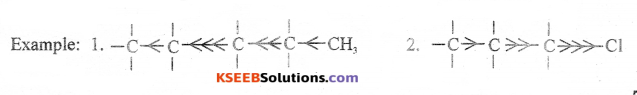Question 36.
(a) An organic compound contains 69% carbon and 4.8% hydrogen, the remainder being oxygen. Calculate the masses of carbon dioxide and water produced when 0.20 g of this substance is subjected to complete combustion.
Given % carbon = 69%, H = 4.8%, w = 0.20g(b) What are nucleophiles? Give an example.
Chemical species having -ve charge reacts with nucleus of an atom/molecule is called nucleophile, e.g: H, CN, H2O, NH3, CP, Br etc.Question 37.
(a) Explain the mechanism of chlorination of methane.
Mechanism of chlorination of methane :
Step I : Chain initiation: Methane and Cl2 heated in UV light, Cl2 undergoes homolytic fission giving chlorine free radicals,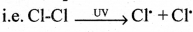Step II: Chain propagation: Cl reacts with methane gives methyl free radical,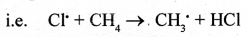Methyl free radical reacts with Cl2 molecule gives C1‘ radical, i.e. CH3 + Cl2 → CH3Cl + Cl’ These are repeated several times.

Step III: Chain termination:
The reaction goes to the end when the free radicals reacts with each other.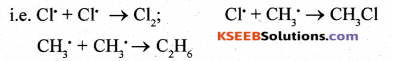(b) Write the Newman’s projections of ethane.